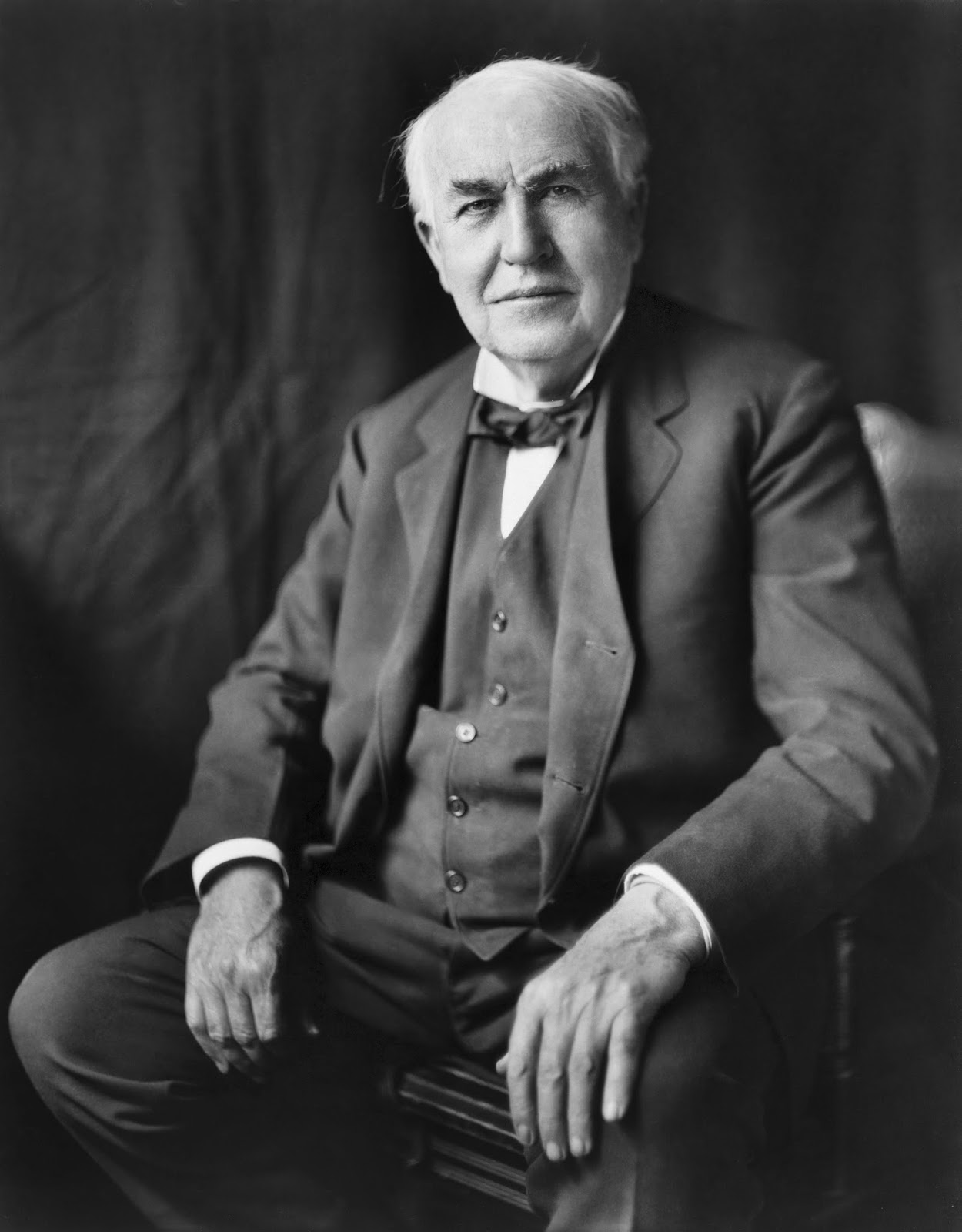## Thursday, January 2, 2014

### 100 lights-puzzle

Another puzzle, because if you haven't figured it out I like puzzles.
Hat tip to Jason Bandlow for showing me this one.

100 lightbulbs are arranged in a 10 by 10 grid.

We have some switches to flip these bulbs. In particular we can flip any 3x3 square (9 lights) and any 5x5 square (25 lights). No wrap-around. Start from everything turned off (this doesn't matter but to keep notation consistent). Can you reach every possible state (any combination of off/on positions of the 100 lights) with these tools?Thomas Edison made it possible to produce 100 light bulbs, so that you can solve puzzles.

1.No. Let V be the vector space over F_2 with basis indexed by the lightbulbs. Each switch corresponds to a vector in V, namely the sum of the corresponding 9 or 25 basis elements, and flipping a switch corresponds to adding the corresponding vector. So the problem is to determine whether these vectors span V. Since there are 36+64=100 switches, this is equivalent to asking whether they are linearly independent. There is a non-trivial linear relation involving six 5x5 squares and ten 3x3 squares, but I'll leave it to anyone reading this to figure out what this is.

A related problem: Is this still true if you replace the on/off lights with lights that take on n different colours, and use switches that change the colours cyclically? I haven't figured this out yet.

1.Yup, you can in fact find a linear relation with four 3x3 squares and four 5x5 squares.

I like the extension problem

2.I suppose for an even number of colours 2k, you can aply your favorite linear relation k times and show that not everything is possible. Any ideas for the odd case?

3.A brute force method would be to construct the 100x100 matrix of the coordinates of the squares in terms of the natural basis, and then look at its determinant. We know it's even, but not whether it's zero.

I haven't figured out what your linear relation is, but if you can lift it to one over Z, you'll have shown the determinant is zero. You can't do this with my relation, and I'd be surprised if you can do it with yours.

One thing you can say: the problem is either solvable for no primes, or all but finitely many.

4.So the relation I know is from Michael Lugo. For purposes of notation I'm going to call the top left bulb (1,1) , the top right bulb (1,10) and the bottum right bulb (10,10). In picture form

(1,1) (1,2) (1,3)....(1,10)
(2,1) (2,2)...........(2,10)
.. .. .. .. ..
.. .. .. ...
(10,1).................(10,10)

The relation I reffered to had the top left hand corner of the 3x3s in:
(1,1) (1,6) (6,1) and (6,6)
the top left hand corners of the 5x5s are in (1,1) (1,4),(4,1) and (4,4)

Applying this when there are infinitly many colours puts every bulb at colour 0, colour 2 or colour 4. It seems that appyling this k times puts these same bulbs at colours 0k,2k and 4k. If there are 2k colours total then these are all colour zero. It similiarly feels llike whichever set of flips you're using they must be applable k times?

What is the proof that it's soluable for all primes or all but finitely many (does it involve some big theorem I don't know?).

2.In that case it's never possible. If you let T(p,q) be the 3x3 square with top left corner at (p,q), and let F(p,q) be the 5x5 square with top left corner at (p,q), then
T(1,1) - T(1,6) - T(6,1) + T(6,6) - F(1,1) + F(1,4) + F(4,1) - F(4,4) = 0
where now we identify squares with elements of the free abelian group on the set of light-bulbs. Thus the determinant is zero, so it's zero modulo all primes (if it were non-zero, it would be non-zero modulo all but finitely many primes p. The 'big theorem' I was using is the fact that this is equivalent to the linear independence over Z/p of the set of vectors whose coordinates are given by the rows). And if you can't do it for p, you can't do it for any integer multiple of p.